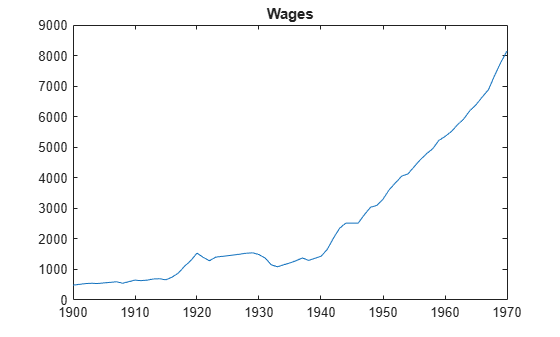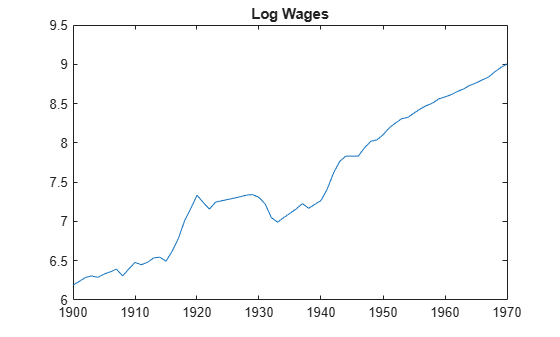# kpsstest

KPSS test for stationarity

## Syntax

``h = kpsstest(y)``
``h = kpsstest(y,Name,Value)``
``````[h,pValue] = kpsstest(___)``````
``````[h,pValue,stat,cValue,reg] = kpsstest(___)``````

## Description

example

``h = kpsstest(y)` returns the logical value (`h`) with the rejection decision from conducting the Kwiatkowski, Phillips, Schmidt, and Shin (KPSS) test for a unit root in the univariate time series `y`.`

example

````h = kpsstest(y,Name,Value)` uses additional options specified by one or more `Name,Value` pair arguments. If any `Name,Value` pair argument is a vector, then all `Name,Value` pair arguments specified must be vectors of equal length or length one. `kpsstest(y,Name,Value)` treats each element of a vector input as a separate test, and returns a vector of rejection decisions.If any `Name,Value` pair argument is a row vector, then `kpsstest(y,Name,Value)` returns a row vector. ```

example

``````[h,pValue] = kpsstest(___)``` returns the rejection decision and p-value for the hypothesis test, using any of the input arguments in the previous syntaxes.```

example

``````[h,pValue,stat,cValue,reg] = kpsstest(___)``` additionally returns the test statistic, critical value, and a structure of regression statistics for the hypothesis test.```

## Examples

collapse all

Reproduce the first row of the second half of Table 5 in Kwiatkowski et al., 1992.

Load the Nelson-Plosser Macroeconomic series data set.

`load Data_NelsonPlosser`

Linearize the real gross national product series (RGNP).

`logGNPR = log(DataTable.GNPR);`

Assess the null hypothesis that the series is trend stationary over a range of lags.

```lags = (0:8)'; [~,pValue,stats] = kpsstest(logGNPR,'Lags',lags,'Trend',true); results = [lags pValue stats]```
```results = 9×3 0 0.0100 0.6299 1.0000 0.0100 0.3367 2.0000 0.0100 0.2421 3.0000 0.0169 0.1976 4.0000 0.0276 0.1729 5.0000 0.0401 0.1578 6.0000 0.0484 0.1479 7.0000 0.0589 0.1412 8.0000 0.0668 0.1370 ```

The tests corresponding to 0 $\le$ `lags` $\le$ 2 produce p-values that are less than 0.01. For `lags` $<$ 7, the tests indicate sufficient evidence to suggest that log rGNP is unit root nonstationary (i.e., not trend stationary) at the default 5% level.

Test whether the wage series in the manufacturing sector (1900-1970) has a unit root.

Load the Nelson-Plosser Macroeconomic data set.

```load Data_NelsonPlosser wages = DataTable.WN; T = sum(isfinite(wages)); % Sample size without NaNs sqrtT = sqrt(T) % See Kwiatkowski et al., 1992```
```sqrtT = 8.4261 ```

Plot the wages series.

```plot(dates,wages) title('Wages') axis tight```The plot suggests that the wages series grows exponentially.

Linearize the wages series.

```logWages = log(wages); plot(dates,logWages) title('Log Wages') axis tight```The plot suggests that the log wages series has a linear trend.

Test the hypothesis that the log wages series is a unit root process with a trend (i.e., difference stationary), against the alternative that there is no unit root (i.e., trend stationary). Conduct the test by setting a range of lags around $\sqrt{T}$, as suggested in Kwiatkowski et al., 1992.

`[h,pValue] = kpsstest(logWages,'lags',[7:10])`
```h = 1x4 logical array 0 0 0 0 ```
```pValue = 1×4 0.1000 0.1000 0.1000 0.1000 ```

All tests fail to reject the null hypothesis that the log wages series is trend stationary.

The p-values are larger than 0.1. The software compares the test statistic to critical values and computes p-values that it interpolates from tables in Kwiatkowski et al., 1992.

## Input Arguments

collapse all

Univariate time series, specified as a vector. The last element is the most recent observation.

`NaN`s indicate missing observations, and `kpsstest` removes them from `y`. Removing `NaN`s decreases the effective sample size and can cause an irregular time series.

### Name-Value Pair Arguments

Specify optional comma-separated pairs of `Name,Value` arguments. `Name` is the argument name and `Value` is the corresponding value. `Name` must appear inside quotes. You can specify several name and value pair arguments in any order as `Name1,Value1,...,NameN,ValueN`.

Example: `'alpha',0.1,'lags',0:2` specifies three tests that include 0, 1, and 2 autocovariance lags in the Newey-West estimator of the long-run variance, each conducted at 0.1 level of significance.

Number of autocovariance lags to include in the Newey-West estimator of the long-run variance, specified as the comma-separated pair consisting of `'lags'` and a nonnegative integer or vector of nonnegative integers. Use a vector to conduct multiple tests.

Example: `'lags',0:2`

Data Types: `double`

Indicate whether to include the deterministic trend term δt in the model, specified as the comma-separated pair consisting of `'trend'` and a logical value or a vector of logical values. Use a vector to conduct multiple tests.

Example: `'trend',false`

Data Types: `logical`

Significance levels for the hypothesis tests, specified as the comma-separated pair consisting of `'alpha'` and a scalar or vector. All values of `alpha` must be between 0.01 and 0.10. Use a vector to conduct multiple tests.

Example: `'alpha',0.01`

Data Types: `double`

## Output Arguments

collapse all

Test rejection decisions, returned as a logical value or vector of logical values with a length equal to the number of tests that the software conducts.

• `h = 1` indicates rejection of the trend-stationary null in favor of the unit root alternative.

• `h = 0` indicates failure to reject the trend-stationary null.

Test statistic p-values, returned as a scalar or vector with a length equal to the number of tests that the software conducts. The p-values are right-tail probabilities.

When test statistics are outside tabulated critical values, `kpsstest` returns maximum (`0.10`) or minimum (`0.01`) p-values.

Test statistics, returned as a scalar or vector with a length equal to the number of tests that the software conducts.

`kpsstest` computes test statistics using an ordinary least squares (OLS) regression.

• If you set `'trend',false`, then the software regresses `y` on an intercept.

• Otherwise, the software regresses `y` on an intercept and trend term.

Critical values, returned as a scalar or vector with a length equal to the number of tests that the software conducts. Critical values are for right-tail probabilities.

Regression statistics for ordinary least squares (OLS) estimation of coefficients in the alternative model, returned as a data structure or data structure array with a length equal to the number of tests that the software conducts.

Each data structure has the following fields.

FieldDescription
`num`Length of input series with `NaN`s removed
`size`Effective sample size, adjusted for lags
`names`Regression coefficient names
`coeff`Estimated coefficient values
`se`Estimated coefficient standard errors
`Cov`Estimated coefficient covariance matrix
`tStats`t statistics of coefficients and p-values
`FStat`F statistic and p-value
`yMu`Mean of the lag-adjusted input series
`ySigma`Standard deviation of the lag-adjusted input series
`yHat`Fitted values of the lag-adjusted input series
`res`Regression residuals
`DWStat`Durbin-Watson statistic
`SSR`Regression sum of squares
`SSE`Error sum of squares
`SST`Total sum of squares
`MSE`Mean square error
`RMSE`Standard error of the regression
`RSq`R2 statistic
`aRSq`Adjusted R2 statistic
`LL`Loglikelihood of data under Gaussian innovations
`AIC`Akaike information criterion
`BIC`Bayesian (Schwarz) information criterion
`HQC`Hannan-Quinn information criterion

collapse all

### Kwiatkowski, Phillips, Schmidt, and Shin (KPSS) Test

Assesses the null hypothesis that a univariate time series is trend stationary against the alternative that it is a nonstationary unit root process.

The test uses the structural model:

`$\begin{array}{l}{y}_{t}={c}_{t}+\delta t+{u}_{1t}\\ {c}_{t}={c}_{t-1}+{u}_{2t},\end{array}$`

where

• δ is the trend coefficient.

• u1t is a stationary process.

• u2t is an independent and identically distributed process with mean 0 and variance σ2.

The null hypothesis is that σ2 = 0, which implies that the random walk term (ct) is constant and acts as the model intercept. The alternative hypothesis is that σ2 > 0, which introduces the unit root in the random walk.

The test statistic is

`$\frac{\sum _{t=1}^{T}{S}_{t}^{2}}{{s}^{2}{T}^{2}},$`

where

• T is the sample size.

• s2 is the Newey-West estimate of the long-run variance.

• ${S}_{t}={e}_{1}+{e}_{2}+\dots {\text{+e}}_{t}.$

## Tips

• In order to draw valid inferences from the KPSS test, you should determine a suitable value for `'lags'`. These two methods determine a suitable number of lags:

• Begin with a small number of lags and then evaluate the sensitivity of the results by adding more lags.

• Kwiatkowski et al.  suggest that a number of lags on the order of $\sqrt{T}$, where T is the sample size, is often satisfactory under both the null and the alternative.

For consistency of the Newey-West estimator, the number of lags must approach infinity as the sample size increases.

• You should determine the value of `'trend'` by the growth characteristics of the time series. Determine its value with a specific testing strategy in mind.

• If a series is growing, then include a trend term to provide a reasonable comparison of a trend stationary null and a unit root process with drift. `kpsstest` sets `'trend',true` by default.

• If a series does not exhibit long-term growth characteristics, then don’t include a trend term (i.e., set `'trend',false`).

## Algorithms

• `kpsstest` performs a regression to find the ordinary least squares (OLS) fit between the data and the null model.

• Test statistics follow nonstandard distributions under the null, even asymptotically. Kwiatkowski et al.  use Monte Carlo simulations, for models with and without a trend, to tabulate asymptotic critical values for a standard set of significance levels between 0.01 and 0.1. `kpsstest` interpolates critical values and p-values from these tables.

 Hamilton, J. D. Time Series Analysis. Princeton, NJ: Princeton University Press, 1994.

 Kwiatkowski, D., P. C. B. Phillips, P. Schmidt, and Y. Shin. “Testing the Null Hypothesis of Stationarity against the Alternative of a Unit Root.” Journal of Econometrics. Vol. 54, 1992, pp. 159–178.

 Newey, W. K., and K. D. West. “A Simple, Positive Semidefinite, Heteroskedasticity and Autocorrelation Consistent Covariance Matrix.” Econometrica. Vol. 55, 1987, pp. 703–708.